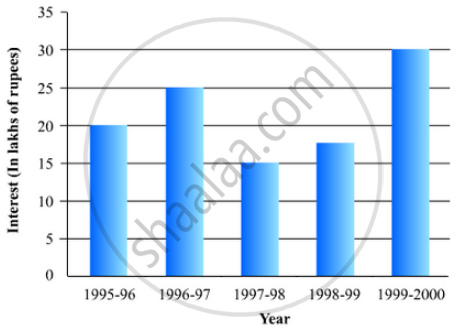# The Following Table Shows the Interest Paid by a Company (In Lakhs): Draw the Bar Graph to Represent the Above Information. - Mathematics

The following table shows the interest paid by a company (in lakhs):

 Year 1995-96 1996-97 1997-98 1998-99 1999-2000 Interest (in lakhs of rupees 20 25 15 18 30

Draw the bar graph to represent the above information.

#### Solution

To represent the given data by a vertical bar graph, we first draw horizontal and vertical axes. Let us consider that the horizontal and vertical axes represent the years and the interests in lakhs of rupees respectively. We have to draw 5 bars of different lengths given in the table.

At first we mark 5 points in the horizontal axis at equal distances and erect rectangles of the same width at these points. The heights of the rectangles are proportional to the interests paid by the company.

The vertical bar graph of the given data is following:Concept: Graphical Representation of Data
Is there an error in this question or solution?

#### APPEARS IN

RD Sharma Mathematics for Class 9
Chapter 23 Graphical Representation of Statistical Data
Exercise 23.2 | Q 8 | Page 26

Share